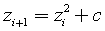The Mandelbrot set

(notes by Roberto Bigoni)

For each complex number c, we can build the sequenceformed by the numbers that, given z0 = 0, are obtained by adding c to the square of the previous term. We shall say that c is the root of the sequence Mc.

The set of the roots c for which Mc does not diverge, that is, such that all the terms zi of Mc have finite absolute value, is called the Mandelbrot set (MS), named after the mathematician Benoit B. Mandelbrot.

It can be shown that if, while developing a sequence Mc, we get a term zi with absolute value ≥ 2, the sequence diverges, then the root c does not belong to MS. This finding implies that, in the complex plane, all of the numbers of MS lie inside a circle of radius 2 centered at the origin. We can call this circle as Mandelbrot Circle (MC).

Since, given a root c, it is physically impossible to calculate all the terms zi of Mc, if a root c is represented in the complex plane by a point inside MC, it is impossible to be certain that c belongs to MS. It can, however, be stated that it does not belongs to this set, if, while developing the sequence Mc, we get soon enough a term zi with absolute value ≥ 2.

So, in order to achieve a quite reliable graphical representation of MS, we can proceed in the following way.

• For each root c we call duration the index of the first of the terms zi of Mc with absolute value ≥ 2.
• We establish the minimum duration that a root c should have to not be excluded from the representation of MS.
• In the complex plane, inside the square with vertices 2 + 2i, -2 + 2i, -2-2i, 2-2i, we choose a suitably dense matrix of evenly spaced points and we represent each of these points with a pixel of a graphic window.
• We calculate the duration of each of these points. If the duration is less than the established minimum, the point does not belong to MS and is represented by a black pixel; otherwise most probably the point belongs to MS and is represented by a white pixel.
• The representation is more reliable the higher the minimum predetermined duration.
• We can get more phantasmagoric graphics by assigning different colors to each point depending on his duration, reserving the white to the probable elements of MS.

The representations that are obtained in this way show interesting properties of MS compared to those of most known figures.

• The frontier of MS is not a continuous line, such as those studied by classical geometry (polygons, circles, conic sections, algebraic, trigonometric, exponential and logarithmic curves, etc.). In fact, it has a non-integer dimension: while in classical geometry lines have dimension 1, surface have dimension2, volumes have dimension 3, etc. this line has a dimensione between 1 and 2.
• MS has an internal homothety: whenever you zoom in on its details you will find figures of the same shape; inside MS there are many tiny MS, inside each of which other more tiny MS, and so on without end.

Mandelbrot called fractals the sets that have these properties.

The following JS application allows you to obtain graphic representations of MS. Clicking on one of them you can select a square and then clicking on the button + you can zoom its content.

last revision: 30/05/2016